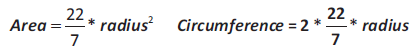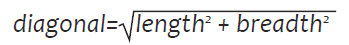Example Programs

Program 

Write a program that outputs the results of the following evaluations based on the number entered by the user.

i. Cube root of the number

ii. Absolute value of the number

iii. Square root of the number

iv. Random numbers between 0 and 1.

Program 

Write a program to input the radius of a circle and find its area and circumference.Program 

Write a program to input three integers and find the sum of their cube roots.

Program 

Write a program to input the area of a square and find its perimeter.

Program 

Write a program to input the length and breadth of a rectangle and find its diagonal.